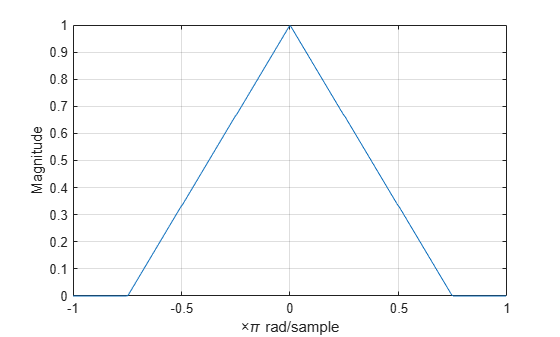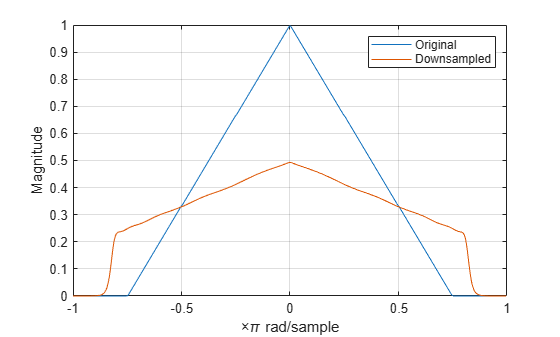# Filtering Before Downsampling

This example shows how to filter before downsampling to mitigate the distortion caused by aliasing. You can use decimate or resample to filter and downsample with one function. Alternatively, you can lowpass filter your data and then use downsample. Create a signal with baseband spectral support greater than $\pi$ radians. Use decimate to filter the signal with a 10th-order Chebyshev type I lowpass filter prior to downsampling.

Create the signal and plot the magnitude spectrum.

f = [0 0.2500 0.5000 0.7500 1.0000]; a = [1.00 0.6667 0.3333 0 0]; nf = 512; b = fir2(nf-1,f,a); Hx = fftshift(freqz(b,1,nf,'whole')); omega = -pi:2*pi/nf:pi-2*pi/nf; plot(omega/pi,abs(Hx)) grid xlabel('\times\pi rad/sample') ylabel('Magnitude')Filter the signal with a 10th-order type I Chebyshev lowpass filter and downsample by 2. Plot the magnitude spectra of the original signal along with the filtered and downsampled signal. The lowpass filter reduces the amount of aliasing distortion outside the interval $\left[-\pi /2,\pi /2\right]$.

y = decimate(b,2,10); Hy = fftshift(freqz(y,1,nf,'whole')); hold on plot(omega/pi,abs(Hy)) legend('Original','Downsampled')